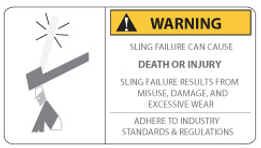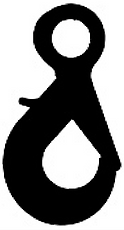# RoundUp™ Round Slings###### HOW TO ORDER INSTRUCTIONS:

Sample part number for a Double Leg, Oblong Link on top, Sling Hooks on bottom and Endless RoundUp™ Roundslings with a maximum capacity of 3600 lbs at 60° and 6' legs:

• Sling configuration:

• Number of legs: (2) - "D" for double

• Top fitting: "O" for oblong

• Bottom fitting: "S" for sling hook

• 3600 lbs. at 60°: leg code - ENR1 or EER1

• Sling length bearing point to bearing point: 4'

• Final Part Number: DOS-ENR1X04

## SINGLE LEG RoundUp™ BRIDLE ROUND SLINGS## MULTI-LEG RoundUp™ BRIDLE ROUND SLINGS*Triple Leg Bridles Available

*Before ordering slings that are going to be used in a chemically active environment, contact Liftex Customer Service to receive a recommendation for the right sling for the right application.

Chemically Active Environments can affect the strength of web slings in varying degrees; ranging from little to total degradation.PROTECT SLINGS FROM CUTTING: Failure to protect sling from cutting may result in damage, injury or death. Contact us to discuss sling protection options.##### Fitting Options

SLING HOOKFOUNDRY HOOKSELF-LOCKING HOOKAdditional items available on request.  Most fittings/hardware available in choice of Domestic or Import.

##### Sling Angle Reduction Factor & Tension Factor
###### METHOD 1 — DETERMINE REDUCTION TO RATED CAPACITY
1. Calculate the Sling to Load Angle (see below — Sling To Load Angle).

2. Determine the associated reduction factor (see chart).

3. Multiply the rated capacity for the basket hitch as indicated on the sling tag by the reduction factor.

4. The result is the safe capacity designation for that sling in that rigging configuration.###### METHOD 2 — DETERMINE INCREASED TENSION/EFFECTIVE WEIGHT OF THE LOAD
1. Calculate the Sling to Load Angle (see below — Sling To Load Angle).

2. Determine the associated tension factor (see chart).

3. Multiply the load weight by the tension factor.

4.  The result is the “Effective Weight” of the load in that rigging configuration — be sure to select a sling with adequate capacity. (A longer sling will increase the Sling to Load angle, thereby reducing the tension factor/effective weight of the load.)

##### HOW TO CALCULATE THE SLING TO LOAD ANGLE
1. You will need two measurements:

• The length of one leg of the sling from where it attaches to the master link to the bearing point of the end fitting. This will be the "Sling Length" dimension in the diagram below.

• The distance between the pick points. Divide this measurement in half and that will be the "Base" dimension in the diagram below.

2. Once you have the Sling Length and Base dimensions you will plug those numbers into the following equation:

•   COS -1 (Base/Sling Length)

• cos -1 is the inverse cosine function and should be available on all smartphones today.

3. First divide the Base dimension by the Sling Length dimension.

4. Next, on the calculator, press the inverse cosine button (it may read as cos-1 or acos) and then type the quotient from the Base/Sling Length equation and then press the equal sign or enter.

5. This will give you the Sling to Load Angle.

6. As an example, let's say that our Sling Length is 16' and the distance between our pick points is 20'.

• First divide 20' in half to get the base dimension: 10'

• Next divide 10 by 16: 0.625

• Then use the inverse cosine function to find the Sling to Load Angle: acos(0.625) = approximately 51°

7. Therefore the Sling to Load Angle for a sling with 16' legs lifting a load with pick points 20' apart is approximately 51°SAFETY NOTE: Use this equation only for lifts where the center of gravity is in the center of the load. If you are lifting a load where the center of gravity is not in the center of the load, then please contact Liftex to receive a recommendation for your application.